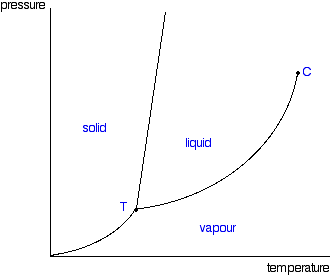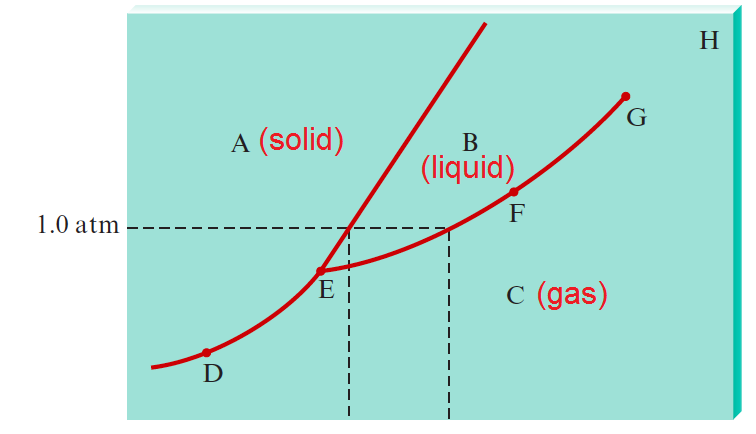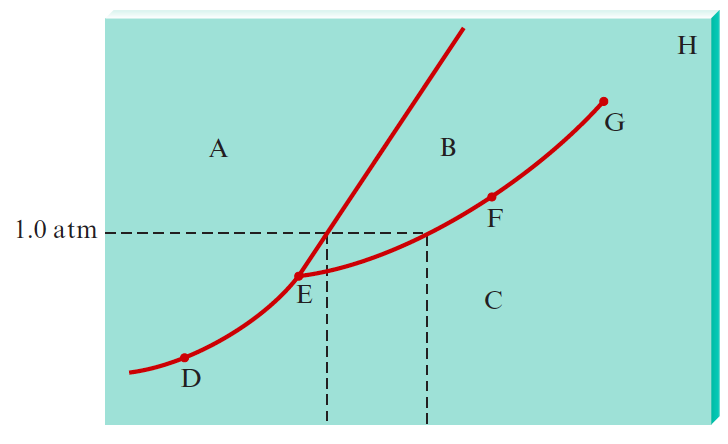# Problem: Consider the phase diagram given below. What phases are present at points A through H? Identify the triple point, normal boiling point, normal freezing point, and critical point. Which phase is denser, solid or liquid?

###### FREE Expert Solution
• A Phase Diagram looks at the transition of matter between the phases of solid, liquid and gas as temperature and pressure are affected.

• Solid phase is usually located in the left of the diagram while gas phase below the diagram. Liquid phase is usually sandwiched between solid and gas phase. Here's a sample of a phase diagram for a pure substance• Comparing this to the given diagram, A appears to be solid, B is liquid and C as gas87% (403 ratings)###### Problem Details

Consider the phase diagram given below. What phases are present at points A through H? Identify the triple point, normal boiling point, normal freezing point, and critical point. Which phase is denser, solid or liquid?